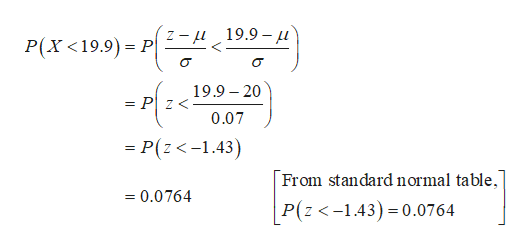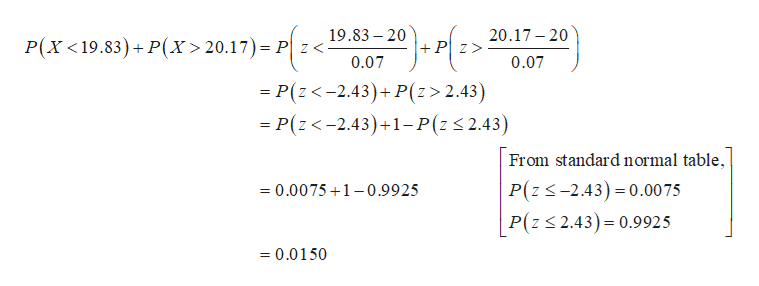# EN>Steel rods are manufactured with a mean length of 20 centimeter (cm). Because of variability in the manufacturing process, the lengths of the rods are approximately normally distributed with a standard deviation of0.07 cm. Complete parts (a) to (d)(a) What proportion of rods has a length less than 19.9 cm?(Round to four decimal places as needed.)(b) Any rods that are shorter than 19.83 cm or longer than 20.17 cm are discarded. What proportion of rods will be discarded?(Round to four decimal places as needed.)(c) Using the results of part (b), if 5000 rods are manufactured in a day, how many should the plant manager expect to discard?(Use the answer from part b to find this answer. Round to the nearest integer as needed.)(d) If an order comes in for 10,000 steel rods, how many rods should the plant manager expect to manufacture if the order states that all rods must be between 19.9 cm and 20.1 cm?(Round up to the nearest integer.)Enter your answer in each of the answer boxes.8:36 PMType here to searchOEH10/20/201919ASUSpausebreakprt scSysraf7f819f1012insertdeletef1f2f3f4f5f6escZtr&backspaceA2#3\$4578070יוPtabWERTY

Questionhelp_outlineImage TranscriptioncloseEN > Steel rods are manufactured with a mean length of 20 centimeter (cm). Because of variability in the manufacturing process, the lengths of the rods are approximately normally distributed with a standard deviation of 0.07 cm. Complete parts (a) to (d) (a) What proportion of rods has a length less than 19.9 cm? (Round to four decimal places as needed.) (b) Any rods that are shorter than 19.83 cm or longer than 20.17 cm are discarded. What proportion of rods will be discarded? (Round to four decimal places as needed.) (c) Using the results of part (b), if 5000 rods are manufactured in a day, how many should the plant manager expect to discard? (Use the answer from part b to find this answer. Round to the nearest integer as needed.) (d) If an order comes in for 10,000 steel rods, how many rods should the plant manager expect to manufacture if the order states that all rods must be between 19.9 cm and 20.1 cm? (Round up to the nearest integer.) Enter your answer in each of the answer boxes. 8:36 PM Type here to search OEH 10/20/2019 19 ASUS pause break prt sc Sysra f7 f8 19 f10 12 insert delete f1 f2 f3 f4 f5 f6 esc Z tr & backspace A 2 # 3 \$ 4 5 7 8 0 7 0יו P tab W E R T Y fullscreen
check_circleExpert Solution
Step 1

a)

The proportion of rods has a length less than 19.9 cm is 0.0764 and it is calculated below:

Let us define the random variable X as the lengths of the rods follows a normal distribution with a mean of 20 and a standard deviation of 0.07.help_outlineImage Transcriptionclose19.9- P(X <19.9) P 19.9 20 = Pz< 0.07 - P(z<-1.43) From standardnormal table, =0.0764 P(z <-1.43) 0.0764 fullscreen
Step 2

b)

The proportion of rods will be discarded is  0.0150 and it is calculated below:

From the given information, the rods will be discarded if length shorter than 19.8...help_outlineImage Transcriptionclose19.83 20 20.17-20 P(X <19.83)P(x> 20.17) Pz< +Pz 0.07 0.07 = P(z<-2.43)+ P(z> 2.43) - P(z<-2.43)+1-P(z 2.43) From standard normal table P(z-2.43) 0.00 75 P(z 2.43) 0.9925 =0.0075 1-0.9925 =0.0150 fullscreen

### Want to see the full answer?

See Solution

#### Want to see this answer and more?

Solutions are written by subject experts who are available 24/7. Questions are typically answered within 1 hour*

See Solution
*Response times may vary by subject and question
Tagged in

### Statistics